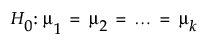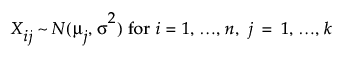Publication date: 11/10/2021

# k Sample Means Calculator

Use the k Sample Means calculator to determine an appropriate sample size for a hypothesis test about two to ten means. Sample size and power are associated with the following hypothesis test:versus the two-sided alternative:

Ha : not all means equal

where: